Courses

# Test: Probability- 1

## 10 Questions MCQ Test Quantitative Aptitude (Quant) | Test: Probability- 1

Description
This mock test of Test: Probability- 1 for Banking Exams helps you for every Banking Exams entrance exam. This contains 10 Multiple Choice Questions for Banking Exams Test: Probability- 1 (mcq) to study with solutions a complete question bank. The solved questions answers in this Test: Probability- 1 quiz give you a good mix of easy questions and tough questions. Banking Exams students definitely take this Test: Probability- 1 exercise for a better result in the exam. You can find other Test: Probability- 1 extra questions, long questions & short questions for Banking Exams on EduRev as well by searching above.
QUESTION: 1

### A die is rolled twice. What is the probability of getting a sum equal to 9?

Solution:

► Total number of outcomes possible when a die is rolled = 6 (∵ any one face out of the 6 faces)

• Hence, total number of outcomes possible when a die is rolled twice, n(S) = 6 x 6 = 36

► E = Getting a sum of 9 when the two dice fall = {(3,6), (4,5), (5,4), (6,3)}

• Hence, n(E) = 4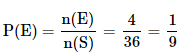QUESTION: 2

### A bag contains 2 yellow, 3 green and 2 blue balls. Two balls are drawn at random. What is the probability that none of the balls drawn is blue?

Solution:

Total number of balls = 2 + 3 + 2 = 7

► Let S be the sample space.

• n(S) = Total number of ways of drawing 2 balls out of 7 = 7C2

► Let E = Event of drawing 2 balls, none of them is blue.

• n(E) = Number of ways of drawing 2 balls from the total 5 (= 7-2) balls = 5C2
(∵ There are two blue balls in the total 7 balls. Total number of non-blue balls = 7 - 2 = 5)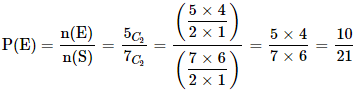QUESTION: 3

### Three coins are tossed. What is the probability of getting at most two tails?

Solution:

► Total number of outcomes possible when a coin is tossed = 2 (∵ Head or Tail)

• Hence, total number of outcomes possible when 3 coins are tossed, n(S) = 2 x 2 x 2 = 8
​(∵ S = {TTT, TTH, THT, HTT, THH, HTH, HHT, HHH})

► E = event of getting at most two Tails = {TTH, THT, HTT, THH, HTH, HHT, HHH}

• Hence, n(E) = 7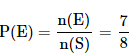QUESTION: 4

When tossing two coins once, what is the probability of heads on both the coins?

Solution:

► Total number of outcomes possible when a coin is tossed = 2 (∵ Head or Tail)

• Hence, total number of outcomes possible when two coins are tossed, n(S) = 2 x 2 = 4
(∵ Here, S = {HH,HT,TH,TT})

► E = event of getting heads on both the coins = {HH}

• Hence, n(E) = 1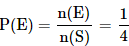QUESTION: 5

What is the probability of getting a number less than 4 when a die is rolled?

Solution:
• Total number of outcomes possible when a die is rolled = 6
(∵ any one face out of the 6 faces) i.e., n(S) = 6
• E = Getting a number less than 4 = {1, 2, 3}
Hence, n(E) = 3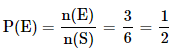QUESTION: 6

A bag contains 4 black, 5 yellow and 6 green balls. Three balls are drawn at random from the bag. What is the probability that all of them are yellow?

Solution:

Total number of balls = 4 + 5 + 6 = 15

► Let S be the sample space.

• n(S) = Total number of ways of drawing 3 balls out of 15 = 15C3

► Let E = Event of drawing 3 balls, all of them are yellow.

• n(E) = Number of ways of drawing 3 balls from the total 5 = 5C3
(∵ there are 5 yellow balls in the total balls)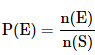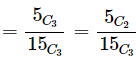[∵ nCr = nC(n-r). So 5C3 = 5C2. Applying this for the ease of calculation]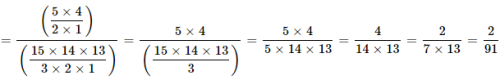QUESTION: 7

One card is randomly drawn from a pack of 52 cards. What is the probability that the card drawn is a face card(Jack, Queen or King)

Solution:
• Total number of cards, n(S) = 52
• Total number of face cards, n(E) = 12 (4 Jacks, 4 Queens, 4 Kings)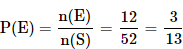QUESTION: 8

A dice is thrown. What is the probability that the number shown in the dice is divisible by 3?

Solution:
• Total number of outcomes possible when a die is rolled, n(S) = 6 (? 1 or 2 or 3 or 4 or 5 or 6)
• E = Event that the number shown in the dice is divisible by 3 = {3, 6}
Hence, n(E) = 2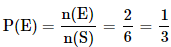QUESTION: 9

John draws a card from a pack of cards. What is the probability that the card drawn is a card of black suit?

Solution:

Total number of cards, n(S) = 52
Total number of black cards, n(E) = 26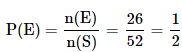QUESTION: 10

There are 15 boys and 10 girls in a class. If three students are selected at random, what is the probability that 1 girl and 2 boys are selected?

Solution:

► Let S be the sample space.

• n(S) = Total number of ways of selecting 3 students from 25 students = 25C3

► Let E = Event of selecting 1 girl and 2 boys

• n(E) = Number of ways of selecting 1 girl and 2 boys

15 boys and 10 girls are there in a class. We need to select 2 boys from 15 boys and 1 girl from 10 girls

Number of ways in which this can be done:
15C2 × 10C1
Hence n(E) = 15C2 × 10C1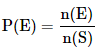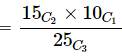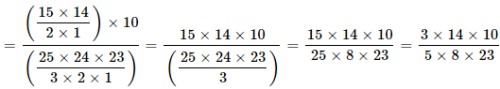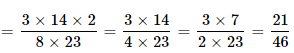Track your progress, build streaks, highlight & save important lessons and more!

### Similar Content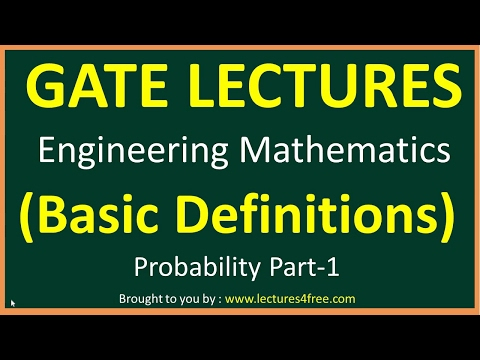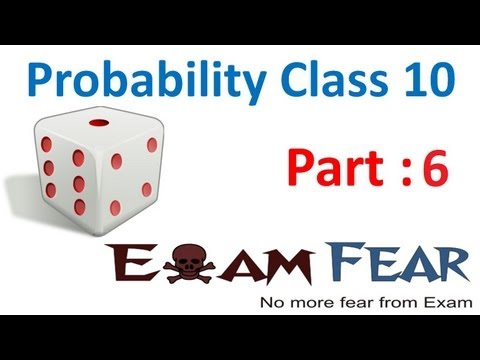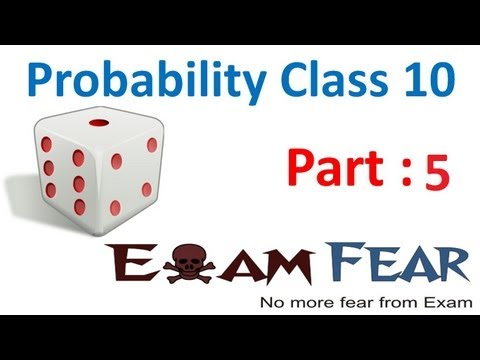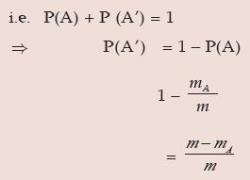### Related tests